Share

Books Shortlist
Your shortlist is empty

# Selina solutions for Class 10 Mathematics chapter 17 - Circles

## Selina ICSE Concise Mathematics for Class 10 (2018-2019)

#### Selina Selina ICSE Concise Mathematics Class 10 (2018-2019)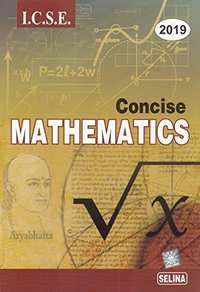## Chapter 17: Circles

17A17B17C17D

#### Chapter 17: Circles Exercise 17A solutions [Page 0]

A chord of length 6 cm is drawn in a circle of radius 5 cm. Calculate its distance from the centre of the circle.

A chord of length 8 cm is drawn at a distance of 3 cm from the centre of a circle. Calculate the radius of the circle.

The radius of a circle is 17.0 cm and the length of perpendicular drawn from its centre to a chord is 8.0 cm. Calculate the length of the chord.

A chord of length 24 cm is at a distance of 5 cm from the centre of the circle. Find the length of the chord of the same circle which is at a distance of 12 cm from the centre.

In the following figure, AD is a straight line. OP ⊥ AD and O is the centre of both the circles. If OA = 34 cm. OB = 20 cm and OP = 16cm; find the length of AB.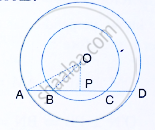O is the centre of a circle of radius 10 cm. P is any point in the circle such that OP = 6 cm. A is the point travelling along the circumference. x is the distance from A to P. what are the least and the greatest values of x in cm? what is the position of the points O, P and A at these values?

In a circle of radius 17 cm, two parallel chords of lengths 30 cm and 16 cm are drawn. Find the distance between the chords, if both the chords are
(i) on the opposite sides of the centre,
(ii) on the same side of the centre.

Two parallel chords are drawn in a circle of diameter 30.0 cm. The length of one chord is 24.0 cm and the distance between the two chords is 21.0 cm; find the length of another chord.

A chord CD of a circle whose centre is O, is bisected at P by a diameter AB.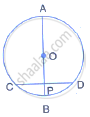Given OA = OB = 15 cm and OP = 9 cm. calculate the length of:
(i) CD (ii) AD (iii) CB

The figure given below, shows a circle with centre O in which diameter AB bisects the chord CD at point E. If CE = ED = 8 cm and EB = 4cm, find the radius of the circle.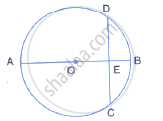The figure shows two concentric circles and AD is a chord of larger circle.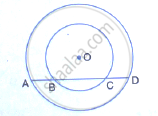Prove that: AB = CD

A straight line is drawn cutting two equal circles and passing through the mid-point M of the line joining their centres O and O’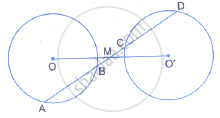Prove that the chords AB and CD, which are intercepted by the two circles are equal.

M and N are the mid-points of two equal chords AB and CD respectively of a circle with centre O. prove that:
(i) ∠BMN  = ∠DNM.
(ii) ∠AMN = ∠CNM.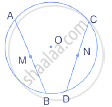In the following figure; P and Q are the points of intersection of two circles with centres O and O’. If straight lines APB and CQD are parallel to O O'; prove that:

(i) O O' = 1/2AB (ii) AB = CD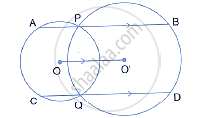Two equal chords AB and CD of a circle with centre O, intersect each other at point P inside the circle, prove that:
(i) AP = CP,
(ii) BP = DP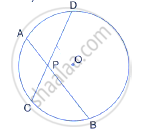In the following figure, OABC is a square. A circle is drawn with O as centre which meets OC
at P and OA at Q. Prove that:
(i) ΔOPA ≅ ΔOQC, (ii) ΔBPC ≅ ΔBQA.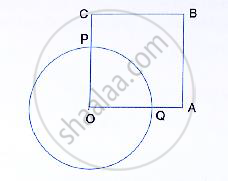The length of common chord of two intersecting circles is 30 cm. If the diameters of these two circles be 50 cm and 34 cm, calculate the distance between their centres.

The line joining the mid-points of two chords of a circle passes through its centre. Prove that the
chords are parallel.

In the following figure, the line ABCD is perpendicular to PQ; where P and Q are the centres of
the circles. Show that:
(i) AB = CD,
(ii) AC = BD.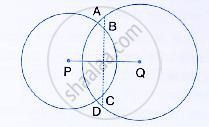AB and CD are two equal chords of a circle with centre O which intersect each other at right
angle at point P. If  OM⊥ AB and ON ⊥ CD; show that OMPN is a square.

#### Chapter 17: Circles Exercise 17B solutions [Page 0]

In the given figure, O is the centre of the circle. ∠OAB and ∠OCB are 30° and 40°
respectively. Find  ∠AOC . Show your steps of working.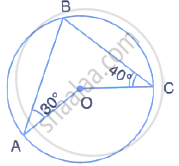In the figure, ∠BAD  = 65° , ∠ABD = 70° , ∠BDC = 45°
(i) Prove that AC is a diameter of the circle
(ii) Find ∠ACB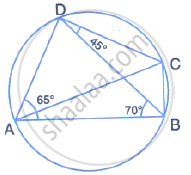Given O is the centre of the circle and ∠AOB  = 70°. Calculate the value of:
(i)  ∠OCA  .
(ii) ∠OCA  .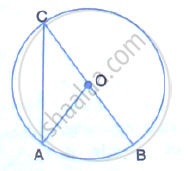In each of the following figures, O is the centre of the circle. Find the values of a, b and c.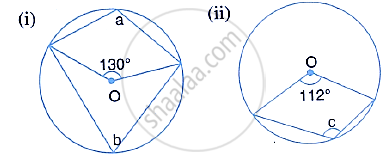In the following figures, O is the centre of the circle. Find the value of a, b, c and d.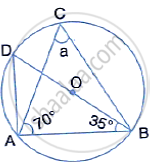In the following figures, O is the centre of the circle. Find the values of a, b, c and d.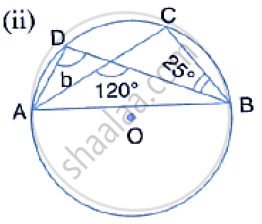In the following figures, O is the centre of the circle. Find the values of a, b, c and d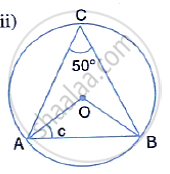.

In the following figures, O is the centre of the circle. Find the values of a, b, c and d.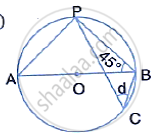In the figure, AB is common chord of the two circle. If AC and AD are diameters; prove that D,
B and C are in a straight line. O1 and O2 are the centres of two circles.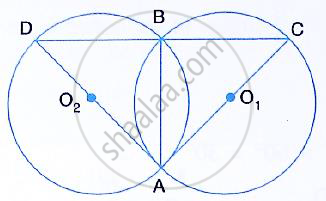In the figure, given below, find: (i) ∠BCD, (ii) ∠ADC, (iii) ∠ABC, Show steps of your working .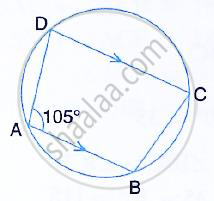In the given figure, O is the centre of the circle. If ∠AOB = 140° and ∠OAC = 50°; Find:
(i) ∠ACB,  (ii) ∠OBC,  (iii) ∠OAB,  (iv) ∠CBA.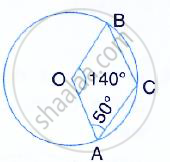Calculate:
(i) ∠CDB, (ii) ∠ABC, (iii) ∠ACB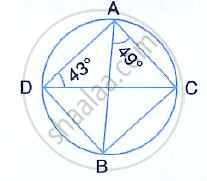In the figure, given below, ABCD is a cyclic quadrilateral in which ∠BAD = 75°; ∠ABD = 58°
and ∠ADC = 77°. Find:

(i) ∠BDC, (ii) ∠BCD, (iii) ∠BCA.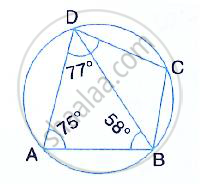In the following figure, O is centre of the circle and ΔABC is equilateral.
Find: (i) ∠ADB, (ii) ∠AEB.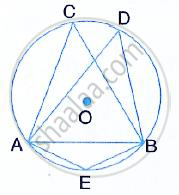Given: ∠CAB = 75° and ∠CBA = 50°. Find the value of ∠DAB + ∠ABD.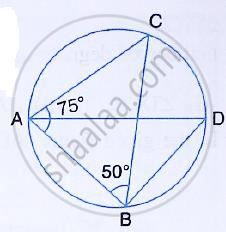ABCD is a cyclic quadrilateral in a circle with centre O. If ∠ADC = 130°; find ∠ BAC.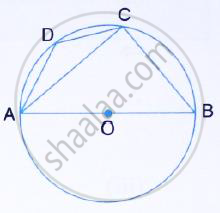In the figure, given below, AOB is a diameter of the circle and ∠AOC = 110°. Find ∠BDC.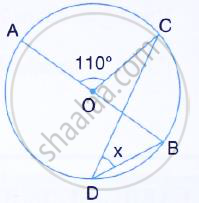In the following figure, O is the centre of the circle,
∠AOB = 60° and ∠BDC = 100° Find ∠OBC.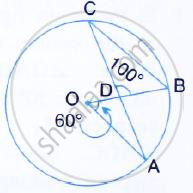ABCD is a cyclic quadrilateral in which  ∠DAC = 27° ; ∠DBA = 50° and ∠ADB = 33°. Calculate:
(i) ∠DBC, (ii) ∠DCB, (iii) ∠CAB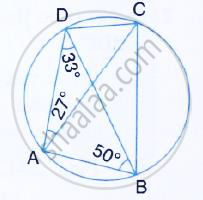In the figure given below, AB is diameter of the circle whose centre is O. given that: ∠ECD =
∠EDC = 32°. Show that ∠COF = ∠CEF.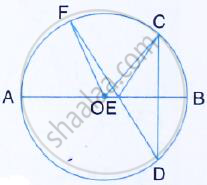In the figure given below, AB and CD are straight lines through the centre O of a circle. If ∠AOC
= 80° and ∠CDE = 40°, Find the number of degrees in:
(i) ∠ DCE, (ii) ∠ABC.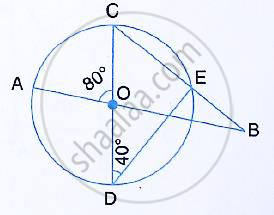In the given figure, AC is a diameter of a circle, whose centre is O. A circle is described on AO
as diameter. AE, a chord of the larger circle, intersects the smaller circle at B. prove that AB =
BE.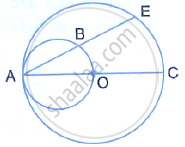In the following figure,
(i) If ∠BAD = 96°, find ∠BCD and ∠BFE.
(ii) Prove that AD is parallel to FE.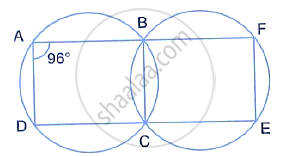Prove that:
the parallelogram, inscribed in a circle, is a rectangle.

Prove that:
the rhombus, inscribed in a circle, is a square.

In the following figure AB = AC. Prove that DECB is an isosceles trapezium.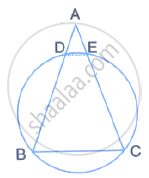Two circles intersect at P and Q. through P diameter PA and PB of the two circles are drawn.
Show that the points A, Q and B are collinear.

ABCD is a quadrilateral inscribed in a circle, having ∠ = 60°; O is the center of the circle. Show that:
∠OBD + ∠ODB
=∠CBD +∠CDB

The figure given below, shows a Circle with centre O.
Given: ∠AOC = a and ∠ABC = b.
(i) Find the relationship between a and b
(ii) Find the measure of angle OAB, is OABC is a parallelogram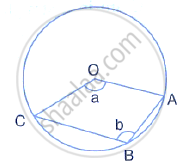Two chords AB and CD intersect at P inside the circle. Prove that the sum of the angles
subtended by the arcs AC and BD at the centre O is equal to twice the angle APC.

In the given figure, RS is a diameter of the circle. NM is parallel to RS and ∠MRS = 29°.
Calculate:
(i) ∠RNM,
(ii) ∠NRM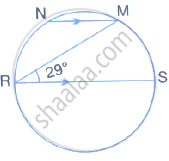In the figure, given alongside, AB ∥ CD and O is the centre of the circle. If ∠ADC = 25°; find
the angle AEB give reasons in support of your answer.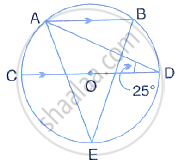Two circle intersect at P and Q. through P, a straight line APB is drawn to meet the circles in A
and B. Through Q, a straight line is drawn to meet the circles at C and D. prove that AC is
parallel to BD.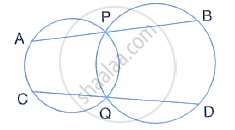ABCD is a cyclic quadrilateral in which AB and DC on being produced, meet at P such that PA
= PD. Prove that AD is parallel to BC.

AB is a diameter of the circle APBR as shown in the figure. APQ and RBQ are straight lines.
Find : (i) ∠PRB,  (ii) ∠PBR,  (iii) ∠BPR.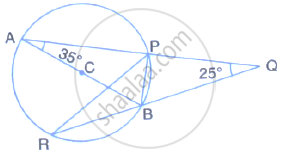In the given figure, SP is bisector of ∠RPT and PQRS is a cyclic quadrilateral. Prove that SQ = SR .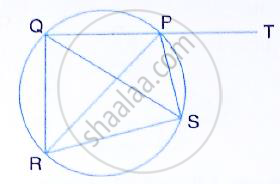In the figure, O is the centre of the circle, ∠AOE = 150°, ∠DAO = 51°. Calculate the sizes of the angles CEB and OCE.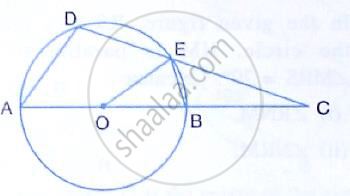In the figure, given below, P and Q are the centres of two circles intersecting at B and C ACD is a straight line. Calculate the numerical value of x .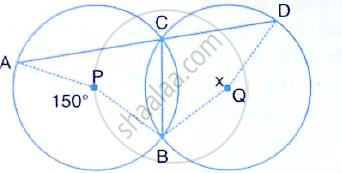The figure shows two circles which intersect at A and B. The centre of the smaller circle is O
and lies on the circumference of the larger circle. Given ∠APB = a°.
Calculate, in terms of a°, the value of:
(i) obtuse ∠AOB,
(ii) ∠ACB
Give reasons for your answers clearly.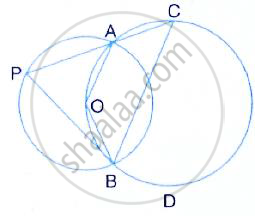In the given figure, O is the centre of the circle and ∠ABC = 55°. Calculate the values of x and y.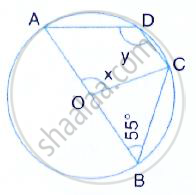In the given figure, A is the centre of the circle, ABCD is a parallelogram and CDE is a straight line.
Prove that : ∠BCD = 2∠ABE .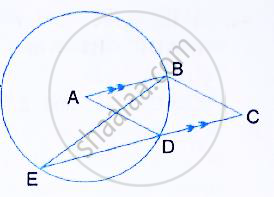ABCD is a cyclic quadrilateral in which AB is parallel to DC and AB is a diameter of the circle. Given ∠BED = 65°; Calculate:
(i) ∠DAB, (ii) ∠BDC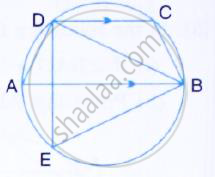In the given figure, AB is a diameter of the circle. Chord ED is parallel to AB and ∠EAB = 63°. Calculate
(i) ∠EBA (ii) ∠BCD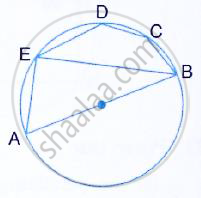The sides AB and DC of a cyclic quadrilateral ABCD are produced to meet at E; the sides DA and CB are produced to meet at F. If ∠BEC = 42° and ∠BAD = 98°; Calculate :
(i) ∠AFB (ii) ∠ADC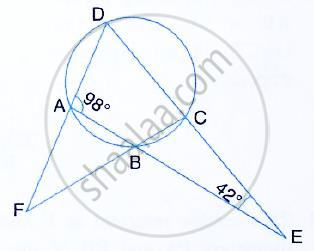In the given figure, AB is a diameter of the circle with centre O. DO is parallel to CB and ∠DCB = 120°.
Calculate:
(i) ∠DAB, (ii) ∠DBA, (iii) ∠DBC, (iv) ∠ADC.
Also show that the ΔAOD is an equilateral triangle .

In the given figure, I is the incentre of ΔABC. BI when produced meets the circumcircle of
ΔABC at D. ∠BAC = 55° and ∠ACB = 65°; calculate:
(i) ∠DCA, (ii) ∠DAC, (iii) ∠DCI, (iv) AIC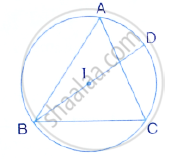A triangle ABC is inscribed in a circle. The bisectors of angles BAC, ABC and ACB meet the circumcircle of the triangle at points P, Q and R respectively. Prove that :
(i) ∠ABC = 2∠APQ,
(ii) ∠ACB = 2∠APR,
(iii) ∠QPR = 90 -1/2 ∠BAC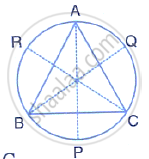Calculate the angles x, y and z if:
x /3= y/4 = z/5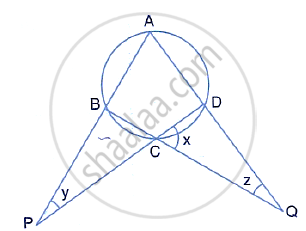In the given figure, AB = AC = CD and ∠ADC = 38°. Calculate:
(i) Angle ABC
(ii) Angle BEC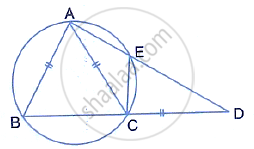In the given figure, AC is a diameter of circle, centre O. Chord BD is perpendicular to AC. Write down the angles p, q and r in terms of x .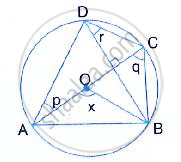In the given figure, AC is the diameter of the circle with centre O. CD and BE are parallel. Angle
∠AOB = 80° and ∠ACE = 10°.
Calculate :
(i) Angle BEC,
(ii) Angle BCD,
(iii) Angle CED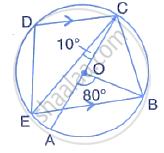In the given figure, AE is the diameter of the circle. Write down the numerical value of ∠ABC+∠CDE. Give reasons for your answer.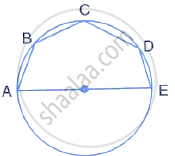In the given figure, AOC is a diameter and AC is parallel to ED. If ∠CBE = 64°, Calculate ∠DEC .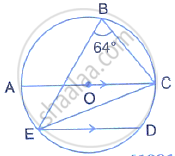Use the given figure to find:
(ii) ∠DQB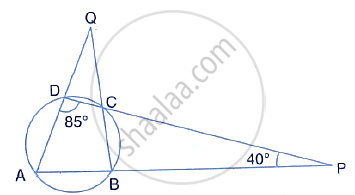In the given figure, AOB is a diameter and DC is parallel to AB. If ∠CAB = x°; find (in terms of x) the values of ;
(i) ∠COB, (ii) ∠DOC, (iii) ∠DAC (iv) ∠ADC.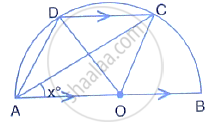In the given figure, AB is the diameter of a circle with centre O. ∠BCD = 130°. Find:
(i) ∠DAB
(ii) ∠DBA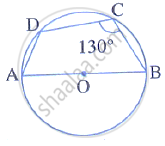In the given figure, PQ is the diameter of the circle whose centre is O. Given ∠ROS = 42°, Calculate ∠RTS.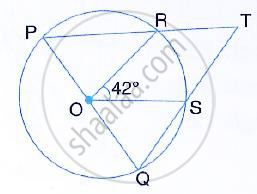In the given figure, PQ is a diameter. Chord SR is parallel to PQ. Given that ∠PQR = 58°,
Calculate:
(i) ∠RPQ, (ii) ∠STP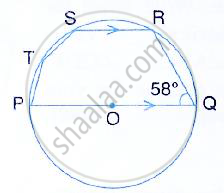AB is the diameter of the circle with centre O. OD is parallel to BC and ∠AOD = 60°. Calculate the numerical values of :
(i) ∠ABD, (ii) ∠DBC, (iii) ∠ADC.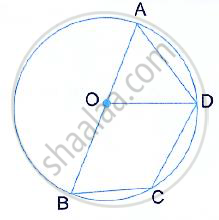In the given figure, the centre O of the small circle lies on the circumference of the bigger circle. If ∠APB = 75° and ∠BCD = 40°, find:
(i) ∠AOB, (ii) ∠ACB, (iii) ∠ABD, (iv) ∠ADB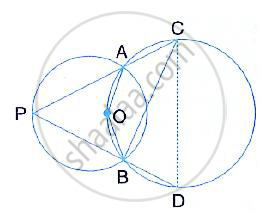In the given figure, ∠BAD = 65°, ∠ABD = 70° and ∠BDC = 45°. Find:
(i) ∠BCD (ii) ∠ACB
Hence, show that AC is a diameter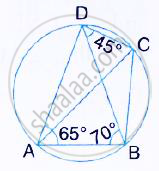In a cyclic quadrilateral ABCD, ∠A : ∠C = 3 : 1 and ∠B : ∠D = 1: 5; Find each angle of the quadrilateral .

The given figure shows a circle with centre O and ∠ABP = 42°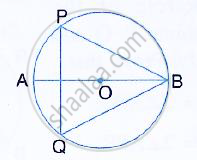Calculate the measure of:
(i) ∠PQB
(ii) ∠QPB + ∠PBQ

In the given figure, M is the centre of the circle. Chords AB and CD are perpendicular to each other.
If ∠MAD = x and ∠BAC = y:
(i) express ∠AMD in terms of x.
(ii) express ∠ABD in terms of y.
(iii) prove that: x = y.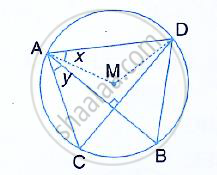In a circle, with centre O, a cyclic quadrilateral ABCD is drawn with AB as a diameter of the circle and CD equal to radius of the circle. If AD and BC produced meet at point P; show that ∠APB = 60°.

#### Chapter 17: Circles Exercise 17C solutions [Page 0]

In the given diagram, chord AB = chord BC.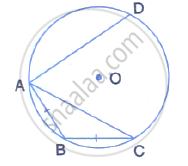(i) what is the relation between arcs AB and BC?
(ii) what is the relation between ∠AOB and ∠BOC?
(iii) If arc AD is greater than arc ABC, then what is the relation between      chords AD and AC?
(iv) If ∠AOB = 50°, find the measure of angle BAC.

In Δ ABC, the perpendicular from vertices A and B on their opposite sides meet (when produced) the circumcircle of the triangle at points D and E respectively. Prove that: arc CD = arc CE

In a cyclic-trapezium, the non-parallel sides are equal and the diagonals are also equal. Prove it.

In the following figure, AD is the diameter of the circle with centre O. chords AB, BC and CD are equal. If ∠DEF = 110°, Calculate:
(i) ∠AEF, (ii) ∠FAB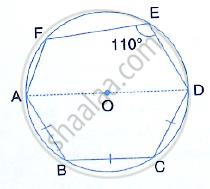In the given figure, if arc AB = arc CD, then prove that the quadrilateral ABCD is an isosceles– trapezium (O is the centre of the circle).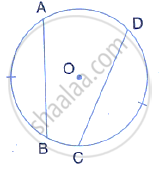In the given figure ABC is an isosceles triangle and O is the centre of its circumcircle. Prove that AP bisects angle BPC .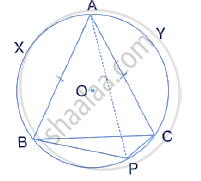If two sides of a cyclic quadrilateral are parallel; prove that:
(i) its other two sides are equal.
(ii) its diagonals are equal.

The given figure shows a circle with centre O. Also, PQ = QR = RS and ∠PTS = 75°. Calculate:
(i) ∠POS,  (ii) ∠QOR,  (iii) ∠PQR.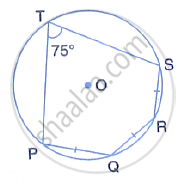In the given figure, AB is a side of a regular six-sided polygon and AC is a side of a regular eight sided polygon inscribed in the circle with centre O. Calculate the sizes of:
(i) ∠AOB,  (ii) ∠ACB  (iii) ∠ABC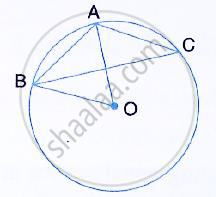In a regular pentagon ABCDE, Inscribed in a circle; find ratio between angle EDA and angle ADC.

In the given figure, AB = BC = CD and ∠ABC = 132 . Calcualte: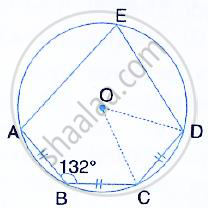(i) ∠AEB,  (ii) ∠AED,  (iii) ∠COD.

In the figure, O is the centre of the circle and the length of arc AB is twice the length of arc BC. If angle AOB = 108°, find: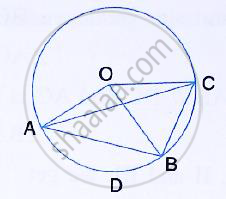(i) ∠CAB  (ii) ∠ADB

The figure shows a circle with centre O. AB is the side of regular pentagon and AC is the side of regular hexagon.
Find the angles of triangle ABC.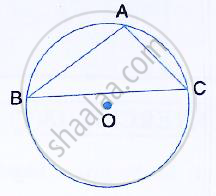In the given figure, BD is a side of a regular hexagon, DC is a side of a regular pentagon and AD is a diameter. Calculate :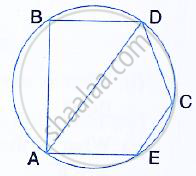(i) ∠ADC (ii) ∠BDA, (iii) ∠ABC, (iv) ∠AEC.

#### Chapter 17: Circles Exercise 17D solutions [Page 0]

In the given circle with diameter AB, find the value of x.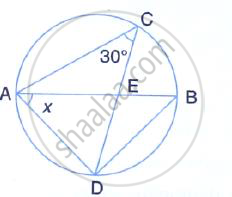In the given figure, O is the centre of the circle with radius 5 cm. OP and OQ are perpendicular to AB and CD respectively. AB = 8 cm and CD = 6 cm. determine the length of PQ.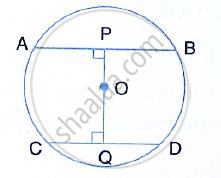The given figure shows two circles with centres A and B; and radii 5 cm and 3 cm respectively, touching each other internally. If the perpendicular bisector of AB meets the bigger circle in P and Q, find the length of PQ.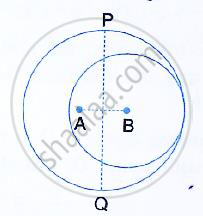In the given figure, ABC is a triangle in which ∠BAC = 30°. Show that BC is equal to the radius of the circumcircle of the triangle ABC, whose centre is O.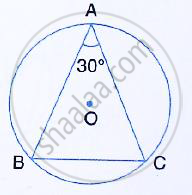Prove that the circle drawn on any one of the equal sides of an isosceles triangle as diameter bisects the base.

In the given figure, chord ED is parallel to diameter AC of the circle. Given ∠CBE = 65°, calculate ∠DEC.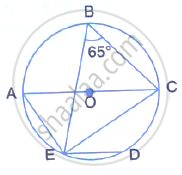Chords AB and CD of a circle intersect each other at point P such that AP = CP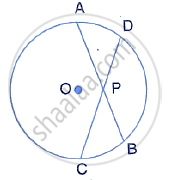Show that: AB = CD

The quadrilateral formed by angle bisectors of a cyclic quadrilateral is also cyclic. Prove it.

If two non-parallel sides of a trapezium are equal, it is cyclic. Prove it. Or An isosceles trapezium is always cyclic. Prove it.

D and E are points on equal sides AB and AC of an isosceles triangle ABC such that AD = AE. Prove that the points B, C, E and D are concyclic.

In the given figure, ABCD is a cyclic quadrilateral. AF is drawn parallel to CB and DA is produced to point E. if ∠ADC = 92°, ∠FAE = 20°; determine ∠BCD. Give reason in support of your answer.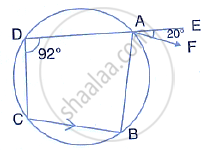If I is the incentre of triangle ABC and AI when produced meets the circumcircle of triangle ABC in point D. If ∠BAC = 66° and ∠ABC = 80°. Calculate :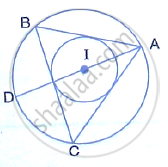(i) ∠DBC  (ii) ∠IBC  (iii) BIC

In the given figure, AB = AD = DC = PB and ∠DBC = x°. determine, in terms of x : (i) ∠ABD, (ii) ∠APB
Hence or otherwise, prove that AP is parallel to DB.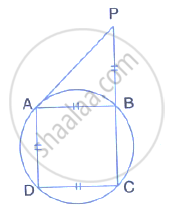In the given figure; ABC, AEQ and CEP are straight lines. Show that ∠APE and ∠CQE are supplementary.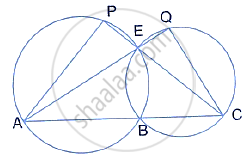In the given figure, AB is the diameter of the circle with centre O.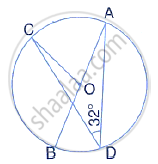If ∠ADC = 32°, find angle BOC

In a cyclic – quadrilateral PQRS, angle PQR = 135°. Sides SP and RQ produced meet at point A : whereas sides PQ and SR produced meet at point B.
If ∠A : ∠B = 2 : 1; find angles A and B.

In the following figure, AB is the diameter of a circle with centre O and CD is the chord with length equal to radius OA.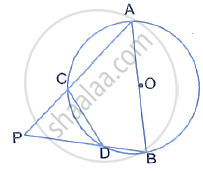Is AC produced and BD produced meet at point P; show that ∠APB = 60°

In the following figure, ABCD is a cyclic quadrilateral in which AD is parallel to BC.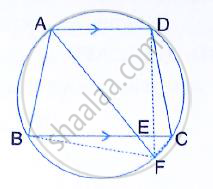If the bisector of angle A meets BC at point E and the given circle at point F, Prove that:
(i) EF = FC (ii) BF = DF

ABCD is a cyclic quadrilateral. Sides AB and DC produced meet at point E; whereas sides BC and AD produced meet at point F.   If ∠DCF : ∠F : ∠E = 3 : 5 : 4, Find the angles of the cyclic quadrilateral ABCD.

The following figure shows a circle with PR as its diameter.
If PQ = 7 cm and QR = 3RS = 6 cm, find the perimeter of the cyclic quadrilateral PQRS.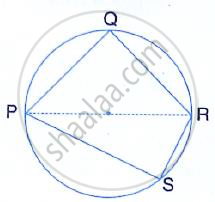In the following figure, AB is the diameter of a circle with centre O.
If chord AC = chord AD, Prove that:
(i) arc BC = arc DB
(ii) AB is bisector of ∠CAD.
Further, if the length of arc AC is twice the length of arc BC, find : (a) ∠BAC (b) ∠ABC

In cyclic quadrilateral ABCD; AD = BC, ∠BAC = 30° and ∠CBD = 70°; find:
(i) ∠BCD (ii) ∠BCA (iii) ∠ABC (iv) ∠ADC

In the given figure, ∠ACE = 43° and ∠CAF = 62° ; Find the values of a, b and c.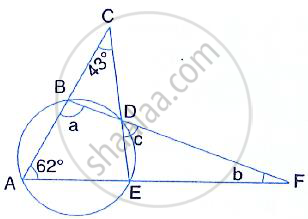In the given figure, AB is parallel to DC ∠BCE = 80° and ∠BAC = 25°.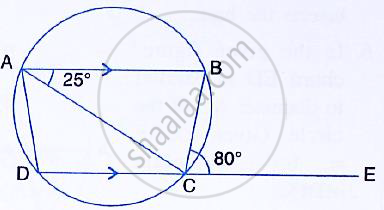Find:

ABCD is a cyclic quadrilateral of a circle with centre O such that AB is a diameter of this circle and the length of the chord CD is equal to the radius of the circle. If AD and BC produced meet at P, Show that APB = 60°

In the figure, given alongside, CP bisects angle ACB. Show that DP bisects angle ADB.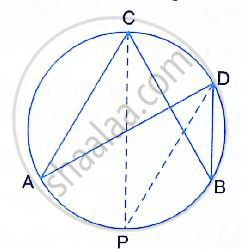In the figure, given below, AD = BC, ∠BAC = 30° and ∠CBD = 70°.
Find:   (i) ∠BCD (ii) ∠BCA (iii) ∠ABC (iv) ∠ADB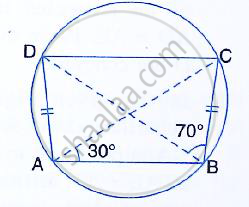In the figure, given below, AB and CD are two parallel chords and O is the centre. If the radius of the circle is 15 cm, fins the distance MN between the two chords of lengths 24 cm and 18 cm respectively.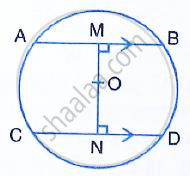## Chapter 17: Circles

17A17B17C17D

#### Selina Selina ICSE Concise Mathematics Class 10 (2018-2019)## Selina solutions for Class 10 Mathematics chapter 17 - Circles

Selina solutions for Class 10 Maths chapter 17 (Circles) include all questions with solution and detail explanation. This will clear students doubts about any question and improve application skills while preparing for board exams. The detailed, step-by-step solutions will help you understand the concepts better and clear your confusions, if any. Shaalaa.com has the CISCE Selina ICSE Concise Mathematics for Class 10 (2018-2019) solutions in a manner that help students grasp basic concepts better and faster.

Further, we at Shaalaa.com are providing such solutions so that students can prepare for written exams. Selina textbook solutions can be a core help for self-study and acts as a perfect self-help guidance for students.

Concepts covered in Class 10 Mathematics chapter 17 Circles are Tangent Properties - If Two Circles Touch, the Point of Contact Lies on the Straight Line Joining Their Centers, Tangent Properties - If a Chord and a Tangent Intersect Externally, Then the Product of the Lengths of Segments of the Chord is Equal to the Square of the Length of the Tangent from the Point of Contact to the Point of Intersection, Tangent Properties - If a Line Touches a Circle and from the Point of Contact, a Chord is Drawn, the Angles Between the Tangent and the Chord Are Respectively Equal to the Angles in the Corresponding Alternate Segments, Areas of Sector and Segment of a Circle, Concept of Circles, Tangent to a Circle, Number of Tangents from a Point on a Circle, Chord Properties - a Straight Line Drawn from the Center of a Circle to Bisect a Chord Which is Not a Diameter is at Right Angles to the Chord, Chord Properties - the Perpendicular to a Chord from the Center Bisects the Chord (Without Proof), Chord Properties - Equal Chords Are Equidistant from the Center, Chord Properties - Chords Equidistant from the Center Are Equal (Without Proof), Chord Properties - There is One and Only One Circle that Passes Through Three Given Points Not in a Straight Line, Arc and Chord Properties - the Angle that an Arc of a Circle Subtends at the Center is Double that Which It Subtends at Any Point on the Remaining Part of the Circle, Arc and Chord Properties - Angles in the Same Segment of a Circle Are Equal (Without Proof), Arc and Chord Properties - Angle in a Semi-circle is a Right Angle, Arc and Chord Properties - If Two Arcs Subtend Equal Angles at the Center, They Are Equal, and Its Converse, Arc and Chord Properties - If Two Chords Are Equal, They Cut off Equal Arcs, and Its Converse (Without Proof), Arc and Chord Properties - If Two Chords Intersect Internally Or Externally Then the Product of the Lengths of the Segments Are Equal, Cyclic Properties.

Using Selina Class 10 solutions Circles exercise by students are an easy way to prepare for the exams, as they involve solutions arranged chapter-wise also page wise. The questions involved in Selina Solutions are important questions that can be asked in the final exam. Maximum students of CISCE Class 10 prefer Selina Textbook Solutions to score more in exam.

Get the free view of chapter 17 Circles Class 10 extra questions for Maths and can use Shaalaa.com to keep it handy for your exam preparation

S